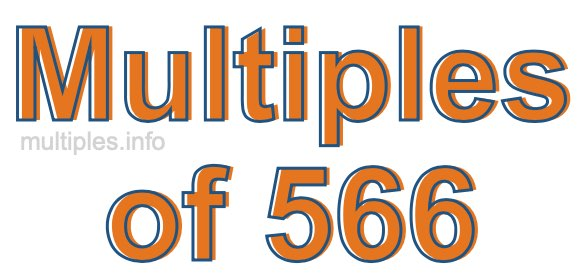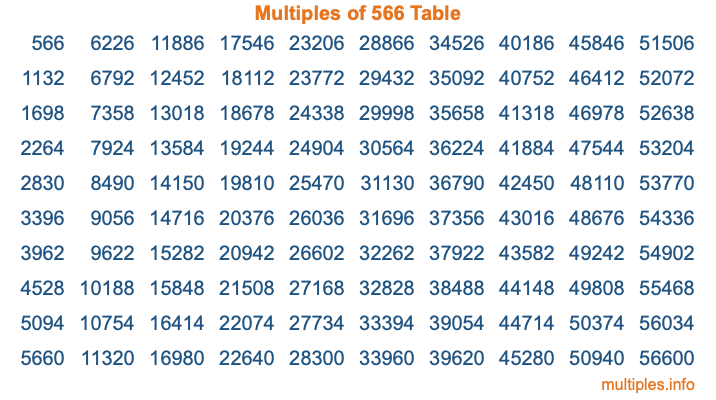Multiples of 566Welcome to the Multiples of 566 page. Here we will first teach you everything you will ever need to know about the multiples of 566, and then give you a study guide summary of everything we taught you to make sure you remember it all. Use this page to look up facts and learn information about the multiples of 566. This page will make you a multiples of five hundred sixty-six expert!

Definition of Multiples of 566
Multiples of 566 are all the numbers that when divided by 566 equal an integer. Each of the multiples of 566 are called a multiple. A multiple of 566 is created by multiplying 566 by an integer.

Therefore, to create a list of multiples of 566, you start with 1 multiplied by 566, then 2 multiplied by 566, then 3 multiplied by 566, and so on for as long as you want. Thus, the list of the first five multiples of 566 is 566, 1132, 1698, 2264, and 2830. To see a larger list of multiples of 566, see the printable image of Multiples of 566 further down on this page. We also have a category where you can choose any nth multiple of 566.

Multiples of 566 Checker
The Multiples of 566 Checker below checks to see if any number of your choice is a multiple of 566. In other words, it checks to see if there is any number (integer) that when multiplied by 566 will equal your number. To do that, we divide your number by 566. If the the quotient is an integer, then your number is a multiple of 566.

Is  a multiple of 566?

Least Common Multiple of 566 and ...
A Least Common Multiple (LCM) is the lowest multiple that two or more numbers have in common. This is also called the smallest common multiple or lowest common multiple and is useful to know when you are adding our subtracting fractions. Enter one or more numbers below (566 is already entered) to find the LCM.

Check out our LCM Calculator if you need more details about the Least Common Multiple or if you need the LCM for different numbers for adding and subtraction fractions.

nth Multiple of 566
As we stated above, 566 is the first multiple of 566, 1132 is the second multiple of 566, 1698 is the third multiple of 566, and so on. Enter a number below to find the nth multiple of 566.

th multiple of 566

Multiples of 566 vs Factors of 566
566 is a multiple of 566 and a factor of 566, but that is where the similarities end. All postive multiples of 566 are 566 or greater than 566. All positive factors of 566 are 566 or less than 566.

Below is the beginning list of multiples of 566 and the factors of 566 so you can compare:

Multiples of 566: 566, 1132, 1698, 2264, 2830, etc.

Factors of 566: 1, 2, 283, 566

As you can see, the multiples of 566 are all the numbers that you can divide by 566 to get a whole number. The factors of 566, on the other hand, are all the whole numbers that you can multiply by another whole number to get 566.

It's also interesting to note that if a number (x) is a factor of 566, then 566 will also be a multiple of that number (x).

Multiples of 566 vs Divisors of 566
The divisors of 566 are all the integers that 566 can be divided by evenly. Below is a list of the divisors of 566.

Divisors of 566: 1, 2, 283, 566

The interesting thing to note here is that if you take any multiple of 566 and divide it by a divisor of 566, you will see that the quotient is an integer.

Multiples of 566 Table
Below is an image of the first 100 multiples of 566 in a table. The table is in chronological order, column by column. The first column has the first ten multiples of 566, the second column has the next ten multiples of 566, and so on.The Multiples of 566 Table is also referred to as the 566 Times Table or Times Table of 566. You are welcome to print out our table for your studies.

Negative Multiples of 566
Although not often discussed or needed in math, it is worth mentioning that you can make a list of negative multiples of 566 by multiplying 566 by -1, then by -2, then by -3, and so on, to get the following list of negative multiples of 566:

-566, -1132, -1698, -2264, -2830, etc.

Multiples of 566 Summary
Below is a summary of important Multiples of 566 facts that we have discussed on this page. To retain the knowledge on this page, we recommend that you read through the summary and explain to yourself or a study partner why they hold true.

There are an infinite number of multiples of 566.

A multiple of 566 divided by 566 will equal a whole number.

566 divided by a factor of 566 equals a divisor of 566.

The nth multiple of 566 is n times 566.

The largest factor of 566 is equal to the first positive multiple of 566.

566 is a multiple of every factor of 566.

566 is a multiple of 566.

A multiple of 566 divided by a divisor of 566 equals an integer.

566 divided by a divisor of 566 equals a factor of 566.

Any integer times 566 will equal a multiple of 566.

Multiples of a Number
Here you can get the multiples of another number, all with the same attention to detail as we did for multiples of 566 on this page.

Multiples of
Multiples of 567
Did you find our page about multiples of five hundred sixty-six educational? Do you want more knowledge? Check out the multiples of the next number on our list!